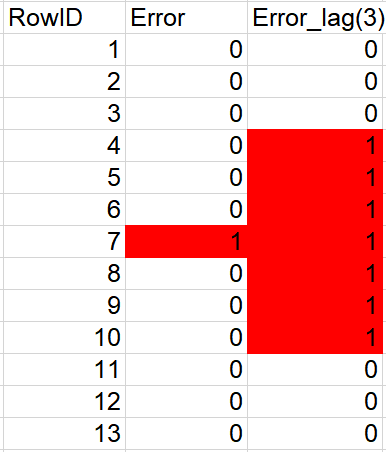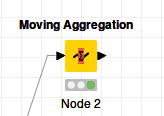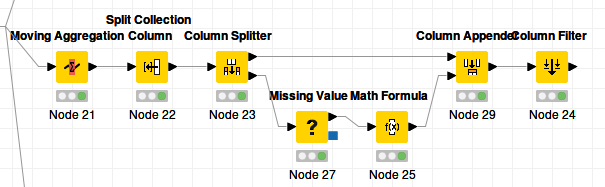# Formula based on previous and following rows

Hi,

I have a column which says if there are errors in each row. The values will either be 1 or 0.
I want to have another column which says if the current row, the previous 3 rows or the next 3 rows have errors. The result will be 1 or 0.

ExampleIn this example the Error_Lag(3) column does what I want. Row 4 to 10 = 1 because row 7 = 1.

I was able to achieve this by:
(1) using the Lag Column node, and lagging for the last 3 rows
(2) sorting the rows in descending order
(3) again use the Lag Column node, and lagging for the last 3 rows
(4) use a column expression node with a big if function.

Previous and next rows.knwf (34.4 KB)

THE QUESTION:
This solution doesn’t seem very elegant. I am wondering if there was a better way to have done this. In Alteryx I could do this using a single node (the Multi-Row formula). I would like to avoid a solution which requires coding. I have read on other threads other possible solutions using Loops or Moving Aggregates but I don’t know how I could apply those to these solutions.

Would someone please be so kind to show me how I could achieve this using:
(1) Loops
(2) Moving aggregates
(3) Any other elegant solution

Thanks!

Calculating stuff using values in previous or following rows is always a bit of a pain in Knime. Indeed, the lag column node is a solution in some cases, but it can only lag in one direction. In the attached workflow are three different solutions that do not involve coding or the lag column node.

The first is using a Moving Aggregation node to find the maximum value in the Error column over a window of 7:The method above restricts you to the functions that are available as aggregation methods in the Moving aggregation node. Therefore I included the second method, which aggregates the aggregation window into a List and then expands the list into columns. You can then use any node you like to process the values in the window because they are now all on one row. In this specific example I use the Math Formula node.The last example is basically the same thing uses a Window Loop instead:

The workflow: KNIME_project.knwf (44.7 KB)

Best
Aswin

4 Likes

Hi @Aswin

Thanks for the reply! Your solution is genius. I haven’t fully gotten my head around the solution but I can see I need more practice with loops.

Cheers